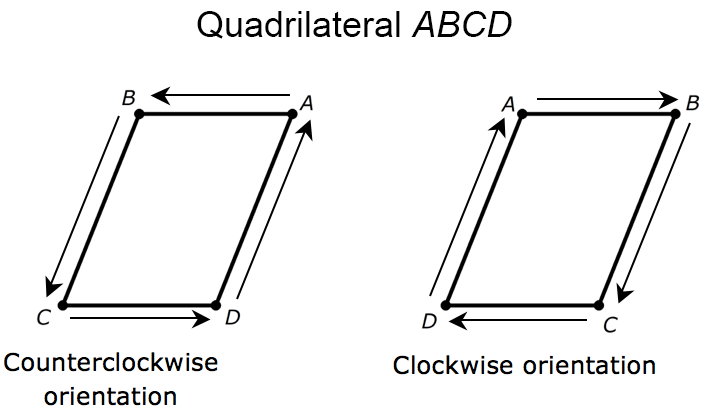Orientation of Vertices

My Definition

Key Characteristics

The following are true about orientation of vertices:

• It is determined by the order in which a figure's vertices are labeled.

• It refers to the sequence of points after a transformation has been applied to a geometric figure.

• The order may be clockwise or counterclockwise.

• It is preserved during these transformations: translations, dilations, and rotations.

• A change in the orientation of the vertices implies a change in the orientation of the figure.

• This definition is used in mathematics.

Example

This is an example of a quadrilateral with counterclockwise and clockwise orientation of vertices.Non-example

This is an example of a change in orientation of position.TEKS: K(6)(E), 8(10)(A)